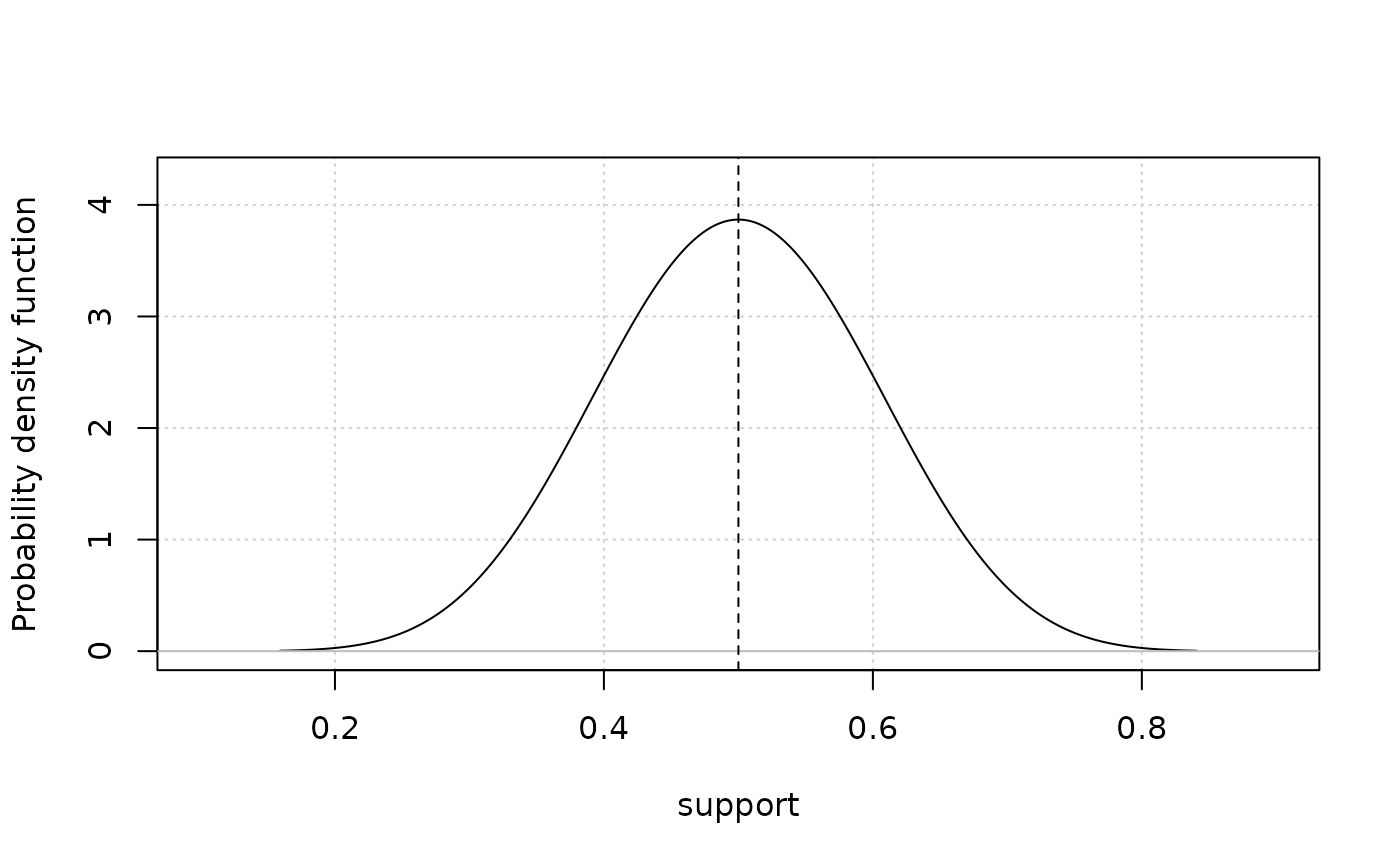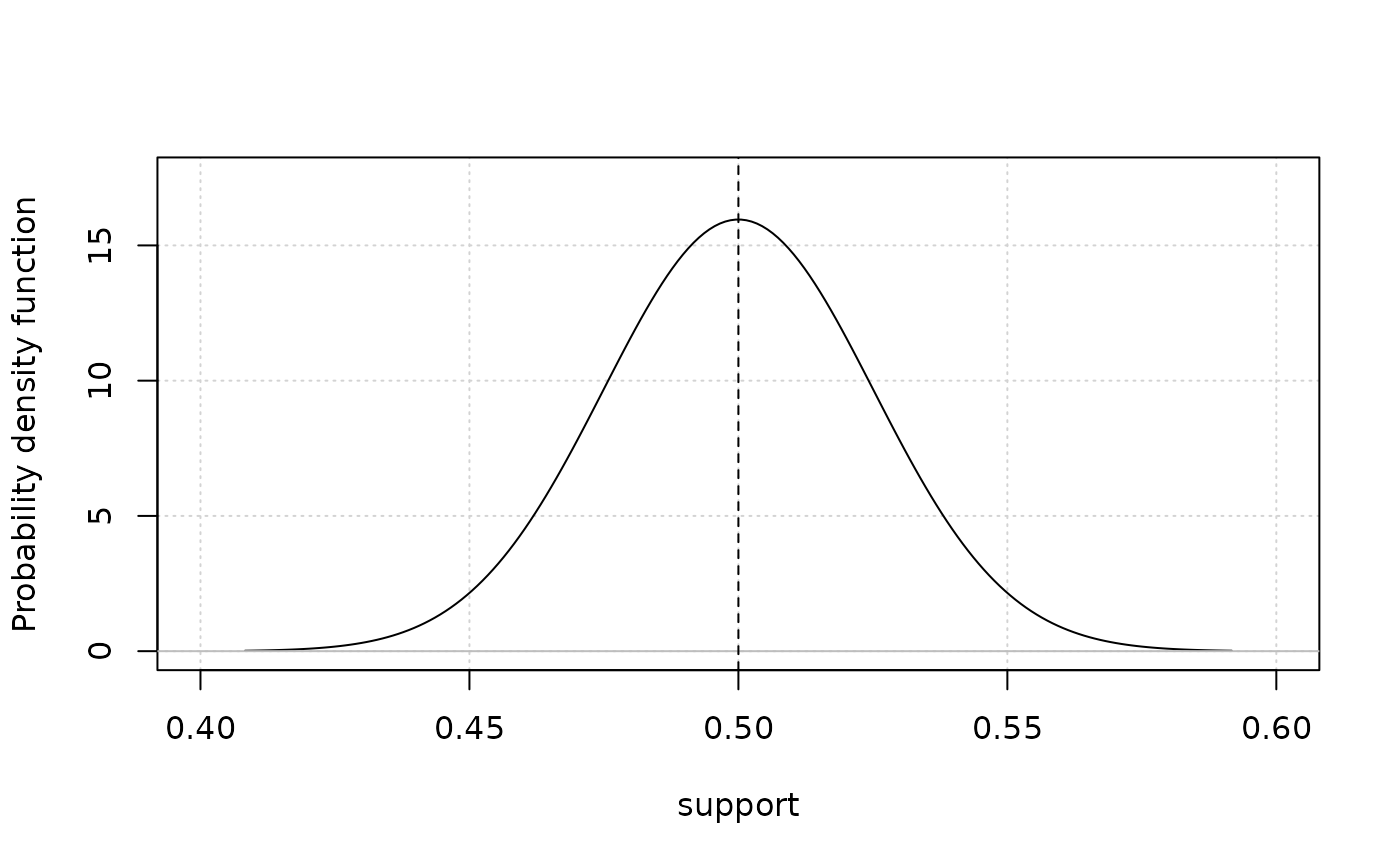Plots the probability distribution function of a beta variable from the mean and standard deviation in either transformed (logit) or untransformed space.

plot_betavar(m, sd, label = NULL, is_logit = FALSE, color = "black")

## Arguments

m

A vector of means of the distribution.

sd

A vector of standard deviations of the distribution.

label

Name of the variable to be used as x-axis label.

is_logit

Logical that indicates whether the means and standard deviations are in logit (TRUE) or normal (FALSE) space.

color

A vector of colors.

## Value

A plot of the probability distribution function. Vertical dotted line indicates mean of distribution. This function can plot multiple curves when multiple means and standard deviations are provided.

plot_lognormalvar plot_steepness

Q. Huynh

## Examples

mu <- 0.5
stddev <- 0.1
plot_betavar(mu, stddev) # mean of plot should be 0.5#logit parameters
mu <- 0
stddev <- 0.1
plot_betavar(mu, stddev, is_logit = TRUE) # mean of plot should be 0.5